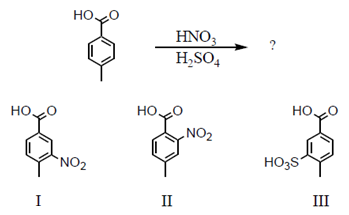# Problem: Which would be the major product(s) of the following reaction? A) I B) II C) III D) I and II in roughly equal amounts E) I and III in roughly equal amounts

###### FREE Expert Solution
100% (191 ratings)
###### Problem Details

Which would be the major product(s) of the following reaction?

A) I

B) II

C) III

D) I and II in roughly equal amounts

E) I and III in roughly equal amountsWhat scientific concept do you need to know in order to solve this problem?

Our tutors have indicated that to solve this problem you will need to apply the EAS: Synergistic and Competitive Groups concept. You can view video lessons to learn EAS: Synergistic and Competitive Groups. Or if you need more EAS: Synergistic and Competitive Groups practice, you can also practice EAS: Synergistic and Competitive Groups practice problems.

What is the difficulty of this problem?

Our tutors rated the difficulty ofWhich would be the major product(s) of the following reactio...as medium difficulty.

How long does this problem take to solve?

Our expert Organic tutor, Jonathan took 1 minute and 35 seconds to solve this problem. You can follow their steps in the video explanation above.

What professor is this problem relevant for?

Based on our data, we think this problem is relevant for Professor Rudd's class at GGC.